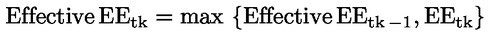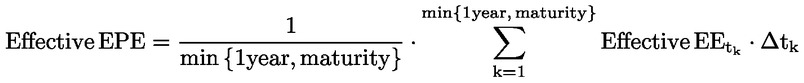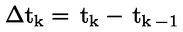In force

Version from: 01/01/2023
Amendments
Search within this legal act

### Article 284 - Exposure value

Article 284

Exposure value

1.
Where an institution is permitted, in accordance with Article 283(1), to use the IMM to calculate the exposure value of some or all transactions mentioned in that paragraph, it shall measure the exposure value of those transactions at the level of the netting set.

The model used by the institution for that purpose shall:

(a)

specify the forecasting distribution for changes in the market value of the netting set attributable to joint changes in relevant market variables, such as interest rates, foreign exchange rates;

(b)

calculate the exposure value for the netting set at each of the future dates on the basis of the joint changes in the market variables.

2.
In order for the model to capture the effects of margining, the model of the collateral value shall meet the quantitative, qualitative and data requirements for the IMM in accordance with this Section and the institution may include in its forecasting distributions for changes in the market value of the netting set only eligible financial collateral as referred to in Articles 197 and 198 and points (c) and (d) of Article 299(2).
3.

The own funds requirement for counterparty credit risk with respect to the CCR exposures to which an institution applies the IMM, shall be the higher of the following:

(a)

the own funds requirement for those exposures calculated on the basis of Effective EPE using current market data;

(b)

the own funds requirement for those exposures calculated on the basis of Effective EPE using a single consistent stress calibration for all CCR exposures to which they apply the IMM.

4.

Except for counterparties identified as having Specific Wrong-Way risk that fall within the scope of Article 291(4) and (5), institutions shall calculate the exposure value as the product of alpha (α) times Effective EPE, as follows:

Exposure value = α · Effective EPE

where:

 α = 1.4, unless competent authorities require a higher α or permit institutions to use their own estimates in accordance with paragraph 9;

Effective EPE shall be calculated by estimating expected exposure (EEt) as the average exposure at future date t, where the average is taken across possible future values of relevant market risk factors.

The model shall estimate EE at a series of future dates t1, t2, t3, etc.

5.

Effective EE shall be calculated recursively as:where:

the current date is denoted as t0;
6.

Effective EPE is the average Effective EE during the first year of future exposure. If all contracts in the netting set mature within less than one year, EPE shall be the average of EE until all contracts in the netting set mature. Effective EPE shall be calculated as a weighted average of Effective EE:where the weightsallow for the case when future exposure is calculated at dates that are not equally spaced over time.
7.
Institutions shall calculate EE or peak exposure measures on the basis of a distribution of exposures that accounts for the possible non-normality of the distribution of exposures.
8.
An institution may use a measure of the distribution calculated by the IMM that is more conservative than α multiplied by Effective EPE as calculated in accordance with the equation in paragraph 4 for every counterparty.
9.

Notwithstanding paragraph 4, competent authorities may permit institutions to use their own estimates of alpha, where:

(a)

alpha shall equal the ratio of internal capital from a full simulation of CCR exposure across counterparties (numerator) and internal capital based on EPE (denominator);

(b)

in the denominator, EPE shall be used as if it were a fixed outstanding amount.

When estimated in accordance with this paragraph, alpha shall be no lower than 1,2.

10.
For the purposes of an estimate of alpha under paragraph 9, an institution shall ensure that the numerator and denominator are calculated in a manner consistent with the modelling methodology, parameter specifications and portfolio composition. The approach used to estimate α shall be based on the institution's internal capital approach, be well documented and be subject to independent validation. In addition, an institution shall review its estimates of alpha on at least a quarterly basis, and more frequently when the composition of the portfolio varies over time. An institution shall also assess the model risk.
11.
An institution shall demonstrate to the satisfaction of the competent authorities that its internal estimates of alpha capture in the numerator material sources of dependency of distribution of market values of transactions or of portfolios of transactions across counterparties. Internal estimates of alpha shall take account of the granularity of portfolios.
12.
In supervising the use of estimates under paragraph 9, competent authorities shall have regard to the significant variation in estimates of alpha that arises from the potential for mis-specification in the models used for the numerator, especially where convexity is present.
13.
Where appropriate, volatilities and correlations of market risk factors used in the joint modelling of market and credit risk shall be conditioned on the credit risk factor to reflect potential increases in volatility or correlation in an economic downturn.